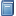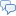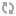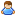BuchempfehlungMikrocomputertechnik mit Controllern der Atmel AVR-RISC-Familie
Umfassend, aber leicht verständlich führt dieses Buch in die Programmierung von ATMEL AVR Mikrocontrollern ein. [Mehr Infos...]FreeBASIC-Chat
Es sindBenutzer im FreeBASIC-Chat online.
(Stand:  )

# fb:porticula NoPaste

## Blurpoint.bas

 Uploader:Eternal_Pain Datum/Zeit: 22.03.2015 14:39:44

```Declare Sub ShowScreensaver() Dim Param as String 'http://www.freebasic-portal.de/tutorials/bildschirmschoner-programmieren-11-s2.html Param = Command(1) If Param = "" Then   Param = "/p" 'End End If 'Wenn ein Parameter angegeben wurde, lösen wir uns das 2. Zeichen heraus (Merke: Das 1. Zeichen war entweder ein Slash oder ein Bindestrich.): Param = Mid(Param, 2, 1) 'Schlussendlich konvertieren wir ihn einfach in einen Kleinbuchstaben: Param = LCase(Param) 'Und jetzt können wir mittels Select-Case ganz einfach entscheiden, was bei welchem Parameter ausgeführt wird: Select Case Param   Case "s","p"     ShowScreensaver()   Case "c"     'ConfigureScreensaver()   Case Else     End End Select randomize timer Function HSV(Byval H as Single) as UInteger     Static as Single  Hue, Saturation, Value, Red, Green, Blue, f, p, q, t     Static as Integer Hs     Hue = ABS(H MOD 360) / 60 : Saturation = 1 : Value = 1     Hs = Hue : f  = Frac(Hue) : p  = Value * (1-Saturation)     q  = Value * (1-(f*Saturation)) : t  = Value * (1-((1-f)*Saturation))     Select Case as Const Hs         Case 0 : Red = Value : Green = t     : Blue = p         Case 1 : Red = q     : Green = Value : Blue = p         Case 2 : Red = p     : Green = Value : Blue = t         Case 3 : Red = p     : Green = q     : Blue = Value         Case 4 : Red = t     : Green = p     : Blue = Value         Case 5 : Red = Value : Green = p     : Blue = q     End Select     Red *= 255 : Green *= 255 : Blue *= 255     Function = RGB(Red,Green,Blue) End Function Sub ScreenSoft()     static as Integer     scrWidth, scrHeight, scrPitch     static as integer ptr scradr     Static as Integer red, green, blue     static as integer ptr bufadr     static as integer bufpitch     static as Integer pix(0 to 4)     static as any ptr bufscr     if bufscr=0 then         ScreenInfo scrWidth, scrHeight,,,scrPitch         scrPitch \= 4         scradr = screenptr         bufscr = imagecreate(scrWidth,scrHeight)         imageinfo bufscr,,,,bufpitch,bufadr         bufpitch \= 4     end if     For y as Integer = 0 to scrHeight - 1     For x as Integer = 0 to scrWidth -1         red = 0 : green = 0 : blue = 0         pix(0) = scradr[x + (y*scrPitch)]         'if pix(0) and &h00FFFFFF Then         If (y >          -1) andalso (x >          0) Then pix(1) = scradr[(x-1) + (y*scrPitch)] Else pix(1) = 0 'left         If (y >           0) andalso (x >         -1) Then pix(2) = scradr[x + ((y-1)*scrPitch)] Else pix(2) = 0 'up middle         If (y >          -1) andalso (x < scrWidth-1) Then pix(3) = scradr[(x+1) + (y*scrPitch)] Else pix(3) = 0 'right         If (y < scrHeight-1) andalso (x >         -1) Then pix(4) = scradr[x + ((y+1)*scrPitch)] Else pix(4) = 0 'down middle         For l as Integer = 0 to 4             red   += lobyte(hiword(pix(l)))             green += hibyte(loword(pix(l)))             blue  += lobyte(loword(pix(l)))         Next l         red shr = 3 'red   \= 5         green shr = 3 'green \= 5         blue shr = 3 'blue  \= 5         bufadr[x + (y*bufPitch)] = rgb(red,green,blue)         'pset bufscr,(x,y),rgb(red,green,blue)         'else         '    pset bufscr,(x,y),pix(0)         'end if     Next x     Next y     put(0,0),bufscr,pset     'imagedestroy(bufscr) End Sub Type ppoint     as Single  dx, dy, ox, oy     as Single  x, y, mx, my     as Integer c, scrWidth, scrHeight, huepal(0 to 359)     Declare Constructor()     Declare Sub DrawPoint() End Type Constructor ppoint()     ScreenInfo scrWidth, scrHeight     x  = rnd * scrWidth : y = rnd * scrHeight     ox = x : oy = y     mx = scrWidth  * 0.03     my = scrHeight * 0.03     dx = rnd * (mx*2) - mx     dy = rnd * (my*2) - my     c  = rnd * 360     for php as integer = 0 to 359         huepal(php) = HSV(php)     next php End Constructor Sub ppoint.DrawPoint()     line (ox,oy)-(x,y),huepal(c)'HSV(c)     ox = x : oy = y     x += dx : y += dy     if x>scrWidth-1  Then dx = - rnd * mx     If x<         0  Then dx =   rnd * mx     if y>scrHeight-1 Then dy = - rnd * my     If y<          0 Then dy =   rnd * my     c += 1     if c >= 360 then c = 0 End Sub Sub ShowScreensaver()     '################################################################     Dim as Integer dskWidth, dskHeight, maxpoint = 25     Dim as ppoint rainbowpoint(0 to maxpoint-1)     screeninfo dskWidth, dskHeight     screenres dskWidth,dskHeight,32,,&h08     'screenres 640,480,32     Dim as Integer lp, brk     Dim as Integer mx, my, mox, moy     dim as string key     setmouse ,,0     sleep 10 'inital-break     dim as integer fps,ofps,fpsa     Dim as double fpstimer = timer     dim as any ptr fpstxtbuffer = imagecreate(100,100)     dim as any ptr fpsoldbuffer = imagecreate(100,100)     Do         key = inkey         getmouse mx, my         if mox = 0 or moy = 0 then mox = mx : moy = my         if mx<>mox or my<>moy or key<>"" then brk = 1         if brk = 0 Then             screenlock                 if fpsa then put (0,0),fpsoldbuffer,pset                 lp = 0                 do                     rainbowpoint(lp).DrawPoint()                     lp += 1                     if multikey(&h01) then                         brk = 1                         exit do                     end if                 loop until lp = maxpoint                 screensoft() 'need more performance!!!!!                 'fps                 fpsa = 1                 get (0,0)-(99,99),fpsoldbuffer                 get (0,0)-(99,99),fpstxtbuffer                 draw string fpstxtbuffer,(5,5),str(ofps)                 put (0,0),fpstxtbuffer,pset             screenunlock         end if         sleep 1         if timer-fpstimer >= 1 then             ofps = fps : fps = 0 : fpstimer = timer         end if         fps += 1     Loop until brk End Sub```